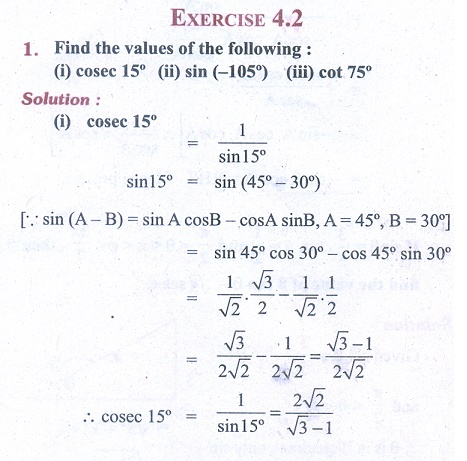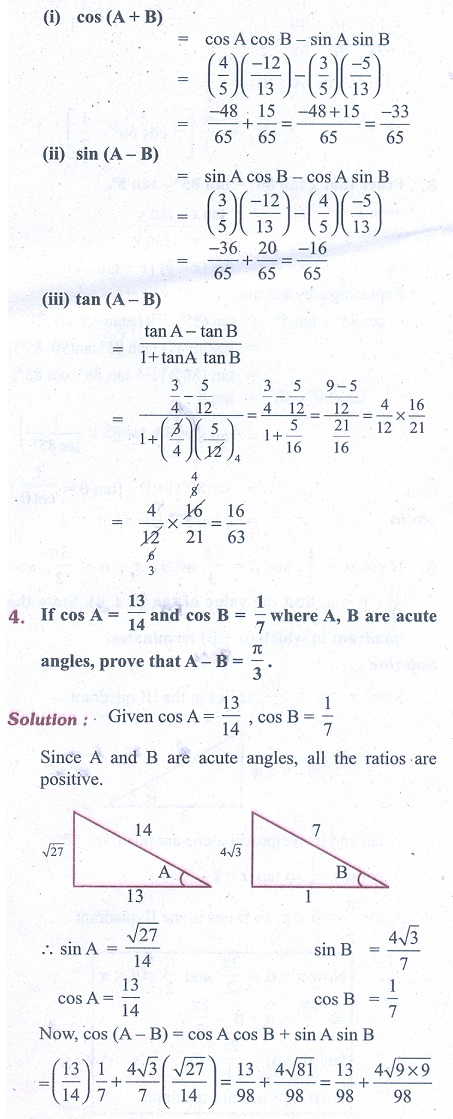Home | | Business Maths 11th std | Exercise 4.2: Trigonometric ratios of compound angles

# Exercise 4.2: Trigonometric ratios of compound angles

Business Mathematics and Statistics Book back answers and solution for Exercise questions - Mathematics: Trigonometry: Trigonometric ratios of compound anglesTags : Problem Questions with Answer, Solution | Trigonometry | Mathematics , 11th Business Mathematics and Statistics(EMS) : Chapter 4 : Trigonometry
Study Material, Lecturing Notes, Assignment, Reference, Wiki description explanation, brief detail
11th Business Mathematics and Statistics(EMS) : Chapter 4 : Trigonometry : Exercise 4.2: Trigonometric ratios of compound angles | Problem Questions with Answer, Solution | Trigonometry | Mathematics